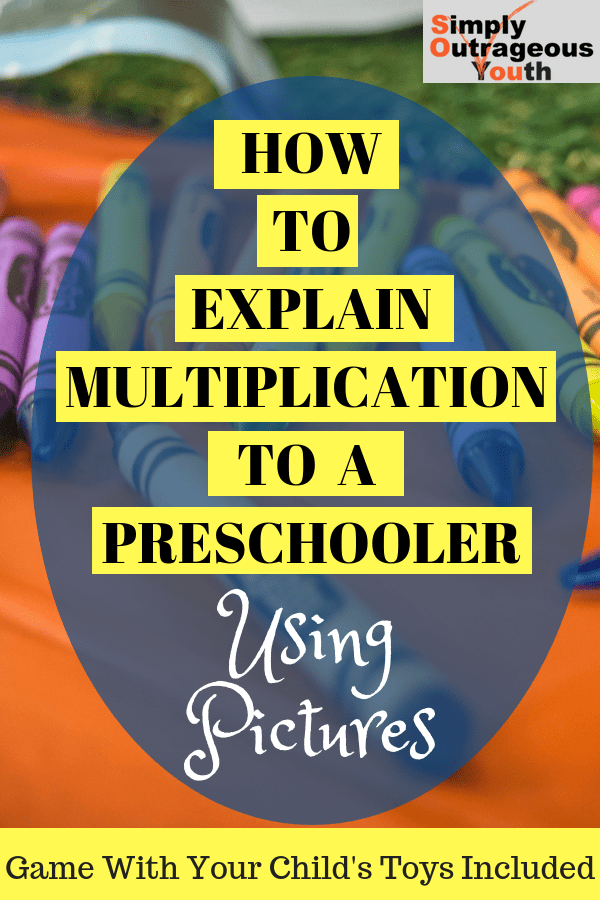###### *This post contains affiliate links, which means I receive a small commission, at no extra cost to you, if you make a purchase using the links.
Some may ask “Why would you explain multiplication to a preschooler?” I will tell you why. One day my son was playing with a math set that included numbers and symbols such as the plus, minus, equal and division signs. He likes to put the numbers in ascending and descending order. While playing, he held up the X (the multiplication sign) and asked “What is this?” When my son asks a question, I usually challenge myself to answer it so he can understand. This time, after hearing the question, I was lost for words. I started to tell him that it’s a multiplication sign and he will learn about it once he gets older. Before uttering these words, I thought a picture would be the best way to explain this concept.

## Go to the Bottom of this Post to Get Access to A Fun Game, Using Action Figures and Stuffed Animals, That Will Explain Multiplication to Young Kids

Here was my process for answering the question, “What is multiplication?” I cut a big piece of craft paper  and taped it to the wall. Then we found crayons and started our quick lesson. Please note: In order to use this explanation, ensure your child is familiar with their numbers, counting, and shapes. With the crayon, I wrote the problem 2×3 =. Then I asked my son to duplicate the problem using the numbers and symbols in his math set. He took the 2, 3, x, and equal sign and made the problem on the floor. Afterwards, I told him the first number(2), tells us to draw two circles on the paper. The second number (3), tell us how many dots to put in each circle. Then I instructed him to do the following…
1. Draw two big circles on the paper.
2. Put three dots in each circle.
3. Count all the dots.Eventually he learned that multiplication is adding a number to itself a certain amount of times. So, 2×3 is the same thing as 3 +3 = 6. We kept going over various examples, until he was able to create a problem and complete it independently. I also explained that it works inversely. You can draw three circles and put two dots in each to solve the problem. This shows that 2+2+2 = 6. He was excited to learn something new and I was proud in my ability to explain this concept to my preschooler!My son solving the problem 8×2 =